Courses

# Test: Stress-Strain Relations & Elastic Constants - 2

## 10 Questions MCQ Test Topicwise Question Bank for Mechanical Engineering | Test: Stress-Strain Relations & Elastic Constants - 2

Description
This mock test of Test: Stress-Strain Relations & Elastic Constants - 2 for Mechanical Engineering helps you for every Mechanical Engineering entrance exam. This contains 10 Multiple Choice Questions for Mechanical Engineering Test: Stress-Strain Relations & Elastic Constants - 2 (mcq) to study with solutions a complete question bank. The solved questions answers in this Test: Stress-Strain Relations & Elastic Constants - 2 quiz give you a good mix of easy questions and tough questions. Mechanical Engineering students definitely take this Test: Stress-Strain Relations & Elastic Constants - 2 exercise for a better result in the exam. You can find other Test: Stress-Strain Relations & Elastic Constants - 2 extra questions, long questions & short questions for Mechanical Engineering on EduRev as well by searching above.
QUESTION: 1

Solution:
QUESTION: 2

### Poisson’s ratio is defined as the ratio of

Solution:

Poisson’s ratio is the ratio of lateral strain to the longitudinal strain.

QUESTION: 3

### Which one of the following materials has Poisson’s ratio more than 1?

Solution:

Poisson’s ratio can not be greater than 0.5.

QUESTION: 4

The maximum values of Poisson’s ratio for an elastic material is

Solution:

Poisson’s ratio lies between 0 to 0.5.
μ = 0.5 for pure elastic rubber
μ = 0.1 to 0.2 for concrete
μ = 0.25 to 0.33 for steel

QUESTION: 5

The relationship between modulus of elasticity (E) and modulus of rigidity (G) is

Solution:
QUESTION: 6

What is the relationship between elastic constants E, G and K?

Solution:

E = 2G(1 + μ)  ......(i)
E = 3K(1 - 2μ)  ......(ii)
Solving (i) and (ii)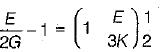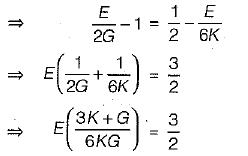⇒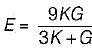QUESTION: 7

In terms of bulk modulus (K) and modulus of rigidity (G), the Poisson’s ratio can be expressed as

Solution:

E = 3K(1 - 2μ)   ......(i)
E = 2G(1 + μ)     .......(ii)
From (i) and (ii)
3K(1 - 2μ) = 2G(1 + μ)
3K - 6μK = 2G + 2μG
3K - 2G = 6μK + 2μG
3K - 2G = 2μ(G + 3K)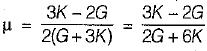QUESTION: 8

What is the relationship between the linear elastic properties Young’s modulus (E), rigidity modulus (G) and bulk modulus (K)?

Solution:

E = 2G(1 + μ)   .......(i)
E = 3K(1 - 2μ)  ........(ii)
Solving (i) and (ii), we get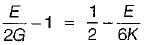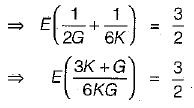⇒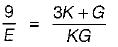∴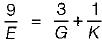QUESTION: 9

A cylindrical bar of 20 mm diameter and 1 m length is subjected to a tensile test. Its longitudinal strain is 4 times that of its lateral strain. If the modulus of elasticity is 2 x 105 N/mm2, then its modulus of rigidity will be

Solution: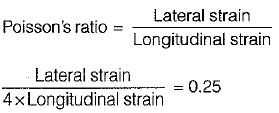But,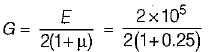= 0.8 x 105 N/mm2

QUESTION: 10

E, G, K and μ represent the elastic modulus, shear modulus, bulk modulus and -Poisson's ratio respectively of a linearly elastic, isotropic and homogeneous material. To express the stress strain relations completely for this material, at least

Solution:

E = 2G(1 + μ)   ...(i)
E = 3K (1 - 2μ)    ...(ii)
In this cae there are four unknown quantities and only two equation are available. Hence any two of the four unknown quantities must be known for complete stress relationship.